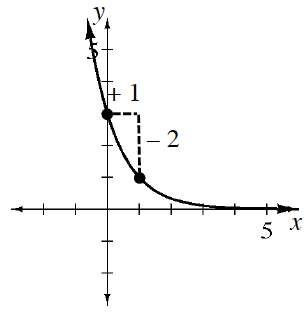### Home > INT1 > Chapter 9 > Lesson 9.1.2 > Problem9-20

9-20.

Use the graph at right to write an equation in $y = ab^x$ form.

The $a$ value is the initial value or $y$-intercept.

The $b$ value is the multiplier. Think of the two given points in a table like you used in finding geometric sequences. What number would you multiply $3$ by to get $1$?